# SAS Criterion in Triangles

SAS Criterion in Triangles

## Introduction to Similarity:

If two triangles are similar it means that:

• All corresponding angle pairs are equal
• All corresponding sides are proportional

However, in order to be sure that two triangles are similar, we do not necessarily need to have information about all sides and all angles.

Think: SAS is a similarity criterion as well as a congruency criterion. What's the difference between the two criteria?

## What is the SAS similarity criterion?

The SAS similarity criterion states that If two sides of one triangle are respectively proportional to two corresponding sides of another, and if the included angles are equal, then the two triangles are similar.

Note the emphasis on the word included. If the equal angle is a non-included angle, then the two triangles may not be similar. Consider the following figure: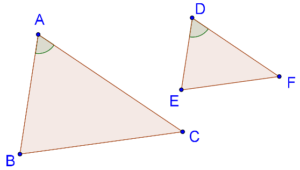It is given that

\begin{align}& \frac{{AB}}{{DE}} = \frac{{AC}}{{DF}} \end{align}

$\angle A = \angle D$

The SAS criterion tells us that $$\Delta ABC$$ ~ $$\Delta DEF$$. Let us see the justification of this.

## Proof of SAS similarity criterion:

Assume that AB > DE.

Given:

1. $$\frac{{DE}}{{AB}} = \frac{{DF}}{{AC}}$$
2. $$\angle D = \angle A$$

To prove:  $$\Delta DEF$$ is similar to $$\Delta ABC$$

Construction:

1. Take a point $$X$$ on $$AB$$ such that $$AX = DE$$
2. Through $$X$$, draw segment $$XY\parallel BC$$, intersecting $$AC$$ at $$Y$$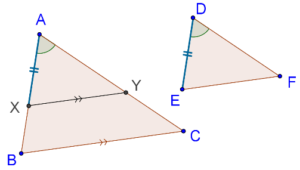Proof: Since $$XY\parallel BC$$, we can note that $$\Delta AXY$$ ~ $$\Delta ABC$$, and thus:

$\frac{{AX}}{{AB}} = \frac{{AY}}{{AC}}....(1)$

Now, we will show that $$\Delta AXY$$ and $$\Delta DEF$$ are congruent. It is given that:

$\frac{{DE}}{{AB}} = \frac{{DF}}{{AC}}....(2)$

Since $$AX = DE$$ (By construction) and from (1) and (2), we have:

$\frac{{DE}}{{AB}} = \frac{{AX}}{{AB}} = \frac{{AY}}{{AC}} = \frac{{DF}}{{AC}}$

Thus,

$AY = DF$

Now, by the SAS congruency criterion,

$\begin{gathered} &\Delta AXY \cong \Delta DEF \hfill \\ \Rightarrow &\Delta AXY \sim \Delta DEF \hfill \\ \end{gathered}$

While we already have, $$\Delta AXY$$ ~ $$\Delta ABC$$,

This means that,

$$\Delta DEF$$ and $$\Delta ABC$$ are similar.

Hence Proved.

Now, Let us see some solved examples and challenge questions.

## Solved Examples:

Example 1: Earlier, you were asked what is the difference between the SAS similarity and congruency criterion?

Solution: In the SAS congruence criterion, you must show that two pairs of sides are equal and their included angles are equal as well. But In the SAS similarity criterion, you must show that two pairs of sides are proportional and their included angles are equal.

⚡Tip:  Two triangles that are similar by the SAS similarity criterion with a scale factor of 1 will be congruent.

Example 2: Consider the following figure: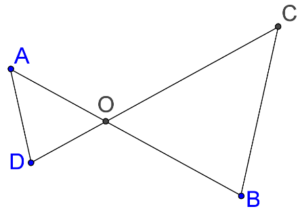Suppose that $$OA \times OB = OC \times OD$$. Show that $$\angle A$$ = $$\angle C$$ and $$\angle B$$ = $$\angle D$$.

Solution: Using the information given to us, we have:

$\frac{{OA}}{{OC}} = \frac{{OD}}{{OB}}$

Thus, two pairs of sides of $$\Delta AOD$$ and $$\Delta COB$$ are respectively proportional.

Also, $$\angle AOD$$ = $$\angle COB$$, because these are vertically opposite angles.

This means that,

$\Delta AOD \sim \Delta COB$

And so,

$$\angle A = \angle C$$ and $$\angle B = \angle D$$

Challenge 1: Consider the same figure:Suppose that $$\angle A = \angle C$$ and $$\angle B = \angle D$$. Show that $$OA \times OB = OC \times OD$$

⚡Tip: It is the converse of example 2.

Example 3: Consider the following figure: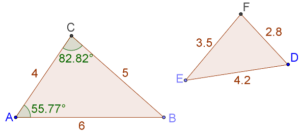Find the value of $$\angle E$$.

Solution: Match the longest side with the longest side and the shortest side with the shortest side and check all three ratios.

We note that the three sides of the two triangles are respectively proportional:

\begin{align}& \left\{ \begin{gathered}\frac{{DE}}{{AB}} = \frac{{4.2}}{6} = 0.7\\ \frac{{DF}}{{AC}} = \frac{{2.8}}{4} = 0.7\\ \frac{{EF}}{{BC}} = \frac{{3.5}}{5} = 0.7 \end{gathered} \right.\\&\quad\frac{{DE}}{{AB}} = \frac{{DF}}{{AC}} = \frac{{EF}}{{BC}} \end{align}

Thus, by SAS similarity criterion, $$\Delta ABC \sim \Delta DEF$$

This means that they are also equi-angular.

Note carefully that the equal angles will be:

$\begin{array}{l} \angle A = \angle D = 55.77^0 \\ \angle C = \angle F = 82.82^0 \\ \angle B = \angle E \end{array}$

Finally,

$\begin{array}{*{20}{l}} {\angle E = \angle B = {{180}^0} - ({{55.77}^0} + {{82.82}^0})} \\ { \Rightarrow \angle E = {{41.41}^0}} \end{array}$

Example 4: Consider two similar triangles, $$\Delta ABC$$ and $$\Delta DEF$$: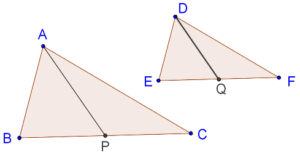$$AP$$ and $$DQ$$ are medians in the two triangles respectively. Show that

$\frac{{AP}}{{BC}} = \frac{{DQ}}{{EF}}$

Solution: Since the two triangles are similar, they are equi-angular.

This means that,

$\angle B = \angle E$

Also,

\begin{align} \frac{{AB}}{{DE}} &= \frac{{BC}}{{EF}}\\ \Rightarrow \quad\frac{{AB}}{{DE}} &= \frac{{BC/2}}{{EF/2}} = \frac{{BP}}{{EQ}} \end{align}

Hence, by the SAS similarity criterion,

$\Delta ABP \sim \Delta DEQ$

Thus, the sides of these two triangles will be respectively proportional, and so:

\begin{align} \frac{{AB}}{{DE}} &= \frac{{AP}}{{DQ}}\\ \Rightarrow \quad\frac{{AP}}{{DQ}} &= \frac{{BC}}{{EF}}\\ \Rightarrow \quad\frac{{AP}}{{BC}} &= \frac{{DQ}}{{EF}} \end{align}

Challenge 2: Consider two similar triangles, $$\Delta ABC$$ and $$\Delta DEF$$:$$AP$$ and $$DQ$$ are medians in the two triangles respectively. Show that $$\Delta APC \sim \Delta DQF$$

⚡Tip: $$P$$ and $$Q$$ are the midpoints of $$BC$$ and $$EF$$.